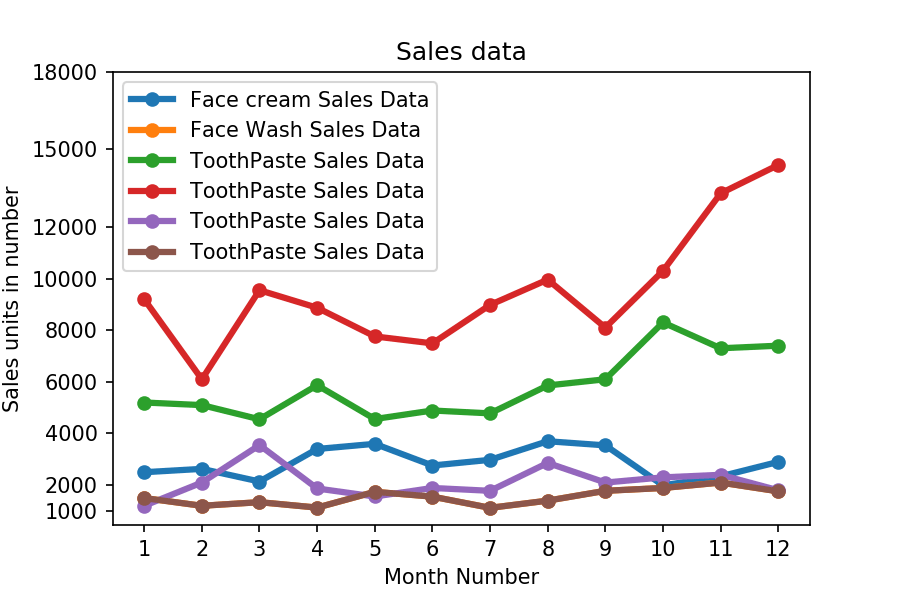Q:

# Read all product sales data and show it using a multiline plot

### Read all product sales data and show it  using a multiline plot

Display the number of units sold per month for each product using multiline plots. (i.e., Separate Plotline for each product ).

The graph should look like this.``````import pandas as pd
import matplotlib.pyplot as plt

monthList  = df ['month_number'].tolist()
faceCremSalesData   = df ['facecream'].tolist()
faceWashSalesData   = df ['facewash'].tolist()
toothPasteSalesData = df ['toothpaste'].tolist()
bathingsoapSalesData   = df ['bathingsoap'].tolist()
shampooSalesData   = df ['shampoo'].tolist()
moisturizerSalesData = df ['moisturizer'].tolist()

plt.plot(monthList, faceCremSalesData,   label = 'Face cream Sales Data', marker='o', linewidth=3)
plt.plot(monthList, faceWashSalesData,   label = 'Face Wash Sales Data',  marker='o', linewidth=3)
plt.plot(monthList, toothPasteSalesData, label = 'ToothPaste Sales Data', marker='o', linewidth=3)
plt.plot(monthList, bathingsoapSalesData, label = 'ToothPaste Sales Data', marker='o', linewidth=3)
plt.plot(monthList, shampooSalesData, label = 'ToothPaste Sales Data', marker='o', linewidth=3)
plt.plot(monthList, moisturizerSalesData, label = 'ToothPaste Sales Data', marker='o', linewidth=3)

plt.xlabel('Month Number')
plt.ylabel('Sales units in number')
plt.legend(loc='upper left')
plt.xticks(monthList)
plt.yticks([1000, 2000, 4000, 6000, 8000, 10000, 12000, 15000, 18000])
plt.title('Sales data')
plt.show()``````